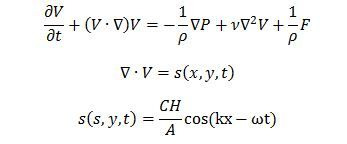# Numerical Wave Tank Construction Use Source Term

Numerical wave flume is now being paid more and more attentions with the rise of wave energy since simulation of wave is the most primary task of studying a wave energy converter by numerical methods. Generally, they can be divided into two types. First, physics mechanism methods such as piston, paddle wave makers Second, pure numerical methods: adding mass source term or momentum source term into governing equations. The second type of wave generating methods stand out for their significant advantages comparing with the first type: first of all, unlike the first type of methods, adding source term into governing equation methods doesn’t require movement of generator which means it will not have the disadvantage of using dynamic mesh. This can avoid error caused by mesh motion and simplified calculation procedure. In addition, pure numerical methods can generate continuous incident wave while not being affected by reflected waves from obstructers.

In our study, numerical wave tank is constructed using commercial CFD software FLUENT using VOF method.  Key equations are given below:where  is the source function, C is the phase velocity, H is wave height, k is wave number and  is the wave frequency. Results showed that our method can simulate linear wave successfully. Figure 1 showed the wave profile when t=50 seconds. Figure 2 compared the time history of wave elevation at x equals one wave length from the beginning of the wave tank and the analytical result. As can be seen, the numerical results agreed with the theory very well.

.
 Figure 1. View of the wave tank at t=50 second. Figure 2.  Comparison between numerical and analytical result.

For more information about the project contact  Dr Qing Xiao (qing.xiao@strath.ac.uk), Lecturer at the Department of Naval Architecture and Marine Engineering at the University of Strathclyde.